I.1.7c

12/24/20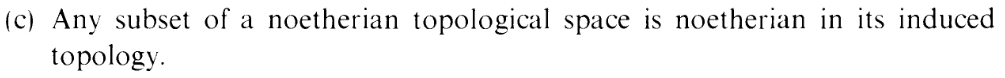Merry fuck you eve. This one is quick

Let Y be a subspace of X, where X is Noetherian. We want to show that Y is Noetherian.

Suppose we have a chain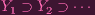(1)

of closed subsets of Y

Note, by the subspace topology, for each i = 1,2,: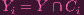(2)

for some Ci closed in X.

For each i, let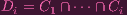(3)

and note that each Di is closed in X.

Then the chain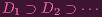(4)

stabilizes since X is Noetherian. So for some n,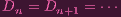(5)

Note: k < i, Y k Y i, so

 Y i = Y i ∩ Y i-1 ∩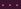∩ Y 1 (6) = (Y ∩ Ci) ∩ (Y ∩ Ci-1) ∩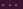∩ (Y ∩ C1) (By (2)) (7) = Y ∩ Ci ∩ Ci-1 ∩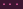∩ C1 (8) = Y ∩ Di (9)

But intersecting Y with (5) yields

 Y ∩ Dn = Y ∩ Dn+1 =(10)Y n = Y n+1 =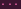(using (9)) (11) (12)

So we're done. WELP, THE EQUATION NUMBERS ARE ALL FORMATTED FUCKED UP, BUT FUCK IT.